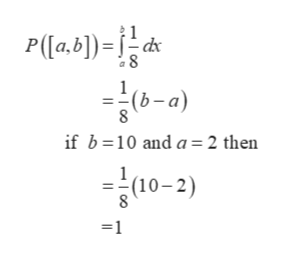# Suppose you choose at random a real number X from the interval [2, 10].(a) Find the density function f(x) and the probability of an event E for this experiment, where E is a subinterval [a, b] of [2, 10].(b) From (a) find the probability that X > 5, that 5 < X < 7, and that X2−12X +35 >0.

Question
63 views

Suppose you choose at random a real number X from the interval [2, 10].

(a) Find the density function f(x) and the probability of an event E for this experiment, where E is a subinterval [a, b] of [2, 10].
(b) From (a) find the probability that X > 5, that 5 < X < 7, and that X2−12X +35 >0.

check_circle

Step 1

First find density function f(x)

Let X follows Uniform distribution

Step 2

X lies in the interval [2, 10]

The density function of X is:

Step 3

The probability of an event E...help_outlineImage TranscriptioncloseP([a.b])=d 8 1 (b-a) 8 if b 10 and a 2 then (10-2) 8 =1 fullscreen

### Want to see the full answer?

See Solution

#### Want to see this answer and more?

Solutions are written by subject experts who are available 24/7. Questions are typically answered within 1 hour.*

See Solution
*Response times may vary by subject and question.
Tagged in

### Other